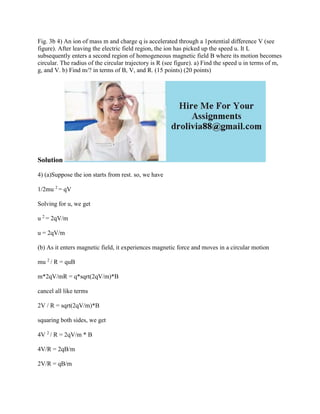Ce diaporama a bien été signalé.
Le téléchargement de votre SlideShare est en cours. ×

# Fig- 3b 4) An ion of mass m and charge q is accelerated through a 1pot.docx

Publicité
Publicité
Publicité
Publicité
Publicité
Publicité
Publicité
Publicité
Publicité
Publicité
PublicitéChargement dans…3
×

## Consultez-les par la suite

1 sur 2 Publicité

# Fig- 3b 4) An ion of mass m and charge q is accelerated through a 1pot.docx

Fig. 3b 4) An ion of mass m and charge q is accelerated through a 1potential difference V (see figure). After leaving the electric field region, the ion has picked up the speed u. It L subsequently enters a second region of homogeneous magnetic field B where its motion becomes circular. The radius of the circular trajectory is R (see figure). a) Find the speed u in terms of m, g, and V. b) Find m/? in terms of B, V, and R. (15 points) (20 points)
Solution
4) (a)Suppose the ion starts from rest. so, we have
1/2mu 2 = qV
Solving for u, we get
u 2 = 2qV/m
u = 2qV/m
(b) As it enters magnetic field, it experiences magnetic force and moves in a circular motion
mu 2 / R = quB
m*2qV/mR = q*sqrt(2qV/m)*B
cancel all like terms
2V / R = sqrt(2qV/m)*B
squaring both sides, we get
4V 2 / R = 2qV/m * B
4V/R = 2qB/m
2V/R = qB/m
m/q = BR/2V
.

Fig. 3b 4) An ion of mass m and charge q is accelerated through a 1potential difference V (see figure). After leaving the electric field region, the ion has picked up the speed u. It L subsequently enters a second region of homogeneous magnetic field B where its motion becomes circular. The radius of the circular trajectory is R (see figure). a) Find the speed u in terms of m, g, and V. b) Find m/? in terms of B, V, and R. (15 points) (20 points)
Solution
4) (a)Suppose the ion starts from rest. so, we have
1/2mu 2 = qV
Solving for u, we get
u 2 = 2qV/m
u = 2qV/m
(b) As it enters magnetic field, it experiences magnetic force and moves in a circular motion
mu 2 / R = quB
m*2qV/mR = q*sqrt(2qV/m)*B
cancel all like terms
2V / R = sqrt(2qV/m)*B
squaring both sides, we get
4V 2 / R = 2qV/m * B
4V/R = 2qB/m
2V/R = qB/m
m/q = BR/2V
.

Publicité
Publicité

### Fig- 3b 4) An ion of mass m and charge q is accelerated through a 1pot.docx

1. 1. Fig. 3b 4) An ion of mass m and charge q is accelerated through a 1potential difference V (see figure). After leaving the electric field region, the ion has picked up the speed u. It L subsequently enters a second region of homogeneous magnetic field B where its motion becomes circular. The radius of the circular trajectory is R (see figure). a) Find the speed u in terms of m, g, and V. b) Find m/? in terms of B, V, and R. (15 points) (20 points) Solution 4) (a)Suppose the ion starts from rest. so, we have 1/2mu 2 = qV Solving for u, we get u 2 = 2qV/m u = 2qV/m (b) As it enters magnetic field, it experiences magnetic force and moves in a circular motion mu 2 / R = quB m*2qV/mR = q*sqrt(2qV/m)*B cancel all like terms 2V / R = sqrt(2qV/m)*B squaring both sides, we get 4V 2 / R = 2qV/m * B 4V/R = 2qB/m 2V/R = qB/m
2. 2. m/q = BR/2V